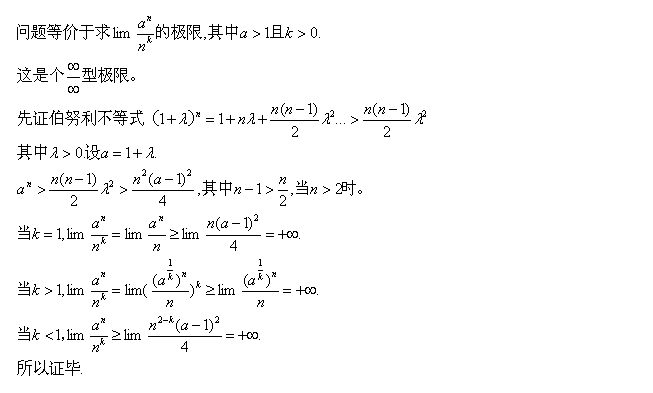• 这是最近一篇的关于多项式复杂度的笔记嘞~大家也在疫情期间加油学习！！！ #9.3.5 Multinomial fitness complexity（多项式复杂度） #subset，子集；intersect，相交 def isSubset(L1, L2): 假定L1和L2是列表 如果...
• 多项式复杂度有弱多项式和强多项式两种，弱多项式就是关于输入长度（ nnn、 mmm 之类的，以及 log值域log 值域log值域）为多项式复杂度，强多项式就是在加减乘除为 O(1)O(1)O(1) 时复杂度关于数据规模为多项式（就是
文章目录前言算法讲解要求无源汇最小费用流做法细节无限小？边权？总结参考资料坑
前言
Ouuan Orz
当然，先说一下弱多项式是啥？
OI 界中叫做 Dinic 和 EK 的那两个最大流算法，把其中的 BFS 改成求最短路，复杂度都是与值域多项式相关的，即复杂度是伪多项式的。
多项式复杂度有弱多项式和强多项式两种，弱多项式就是关于输入长度（ $n$、 $m$ 之类的，以及 $log 值域$）为多项式复杂度，强多项式就是在加减乘除为 $O(1)$ 时复杂度关于数据规模为多项式（就是说跟值域完全无关，只和 $n, m$ 之类的相关，复杂度关于 $n, m$ 之类的为多项式）。
当然，这时$Ouuan$说的。
算法讲解
要求

$st$无入边，$ed$无出边。
可以有负环。

无源汇最小费用流
对于有源汇最小费用最大流的定义我们改一下：
没有$st$和$ed$，同时最小化$\sum\limits_{(i,j)∈E}cost(i,j)*f(i,j)$。
当然，这个时候你可能会好奇这个我们讲的有源汇最小费用最大流有个$der$的关系？
那如果我们从$ed$向$st$连接一条无限小的边，使得流量越多越好，然后后面减掉就行了。
做法
首先，这个流是最小费用当且仅当图中不存在负环，证明和上篇重制版最小费用最大流博客雷同，不予以赘述。
首先明白一个定理：如果把每条边容量乘$2$，则对应流量乘$2$，最小费用乘$2$，因为乘$2$一定不存在负环。
那么接下来就非常简单了，把边权拆成二进制，维护残余网络$G$，一开始$G$中的容量和流量都为$0$，然后二进制从高到低扫描，每一位把所有边的容量和流量乘$2$，但是需要注意，有些边这一位二进制可能为$1$，因此这条边会加入到残余网络中，这就非常的蛋疼了，好的方法是这条边是$(x,y)$，在加入前从$y$跑一遍最短路，如果$d[x]+cost(x,y)<0$，那么就不加入，并且把$y$到$x$的最短路的流量全部减一，当然，如果这条边原本就存在，则直接流量$+1$即可。
至于为什么直接跑最短路即可，因为我们维护的残余网络中一定没有负环啊。
时间复杂度：$O(nm^2\log{U})$，$U$是边中的最大流量。
例题：https://uoj.ac/problem/487
#include<cstdio>
#include<cstring>
#define  N  5100
#define  M  110000
using  namespace  std;
typedef  long  long  LL;
template<class  T>
inline  T  mymin(T  x,T  y){return  x<y?x:y;}
template<class  T>
inline  T  mymax(T  x,T  y){return  x>y?x:y;}
int  n,m;
struct  node
{
int  x,y,next;
LL  c/*表示它们现在现有的流量*/,d;
}a[M];int  len=1,last[N];
LL  cap[N];//表示它们原本的流量
inline  void  ins_node(int  x,int  y,LL  c,LL  d){len++;a[len].x=x;a[len].y=y;a[len].d=d;cap[len]=c;a[len].next=last[x];last[x]=len;}
inline  void  ins(int  x,int  y,LL  c,LL  d){ins_node(x,y,c,d);ins_node(y,x,0,-d);}
//SPFA
LL  d[N];
bool  v[N],vv[N];
void  spfa(int  st,int  ed)
{
memset(d,20,sizeof(d));d[st]=0;
memset(pre,0,sizeof(pre));
memset(v,0,sizeof(v));v[st]=1;
{
v[x]=0;
for(int  k=last[x];k;k=a[k].next)
{
int  y=a[k].y;
if(a[k].c>0  &&  d[y]>d[x]+a[k].d)
{
d[y]=d[x]+a[k].d;
pre[y]=k;
if(!v[y])
{
v[y]=1;
list[tail++]=y;
if(tail==n+1)tail=1;
}
}
}
}
}
LL  cost=0,ans=0,ffuck=0;
void  trash(int  st,int  ed)
{
LL  mmax=0/*,sum=0用来记录st-ed添加的那一条边应该是多少*/,summ=0;
for(int  i=2;i<=len;i++)
{
//		if(a[i].d>0)sum+=a[i].d;
summ+=cap[i];
mmax=mymax(mmax,cap[i]);
}
ins(ed,st,summ,-(LL)999999999);
mmax=mymax(mmax,cap[len-1]);
int  l=1;
while(((LL)1<<l)-1<mmax)l++;

for(int  ll=l;ll>=1;ll--)//从高位到低位开始扫描二进制
{
cost<<=1;
LL  shit=((LL)1<<(ll-1));
memset(vv,0,sizeof(vv));
for(int  i=2;i<=len;i++)
{
a[i].c<<=1;
if(a[i].c)a[i].c+=(cap[i]&shit)>0,vv[i]=1;
}
//对于所有已经存在的边不用扫描
for(int  i=2;i<=len;i++)
{
if(vv[i])continue;
if(cap[i]&shit)//反向边绝对不会进来
{
int  x=a[i].x,y=a[i].y;
spfa(y,x);
if(d[x]+a[i].d<0/*负环!!!!*/)
{
cost+=a[i].d;
x=pre[x];
while(x)
{
cost+=a[x].d;
a[x].c--;a[x^1].c++;
x=pre[a[x].x];
}
a[i^1].c++;
}
else  a[i].c++;
}
}
}
for(int  k=last[st];k;k=a[k].next)
{
if(!(k&1))ans+=cap[k]-a[k].c;
}
printf("%lld %lld\n",ans,cost-(cap[len-1]-a[len-1].c)*a[len-1].d+ffuck);
}
int  main()
{
int  st,ed;
scanf("%d%d%d%d",&n,&m,&st,&ed);
for(int  i=1;i<=m;i++)
{
int  x,y;LL  c,d;scanf("%d%d%lld%lld",&x,&y,&c,&d);
if(x==y)
{
if(d<0)ffuck+=c*d;//自环
}
else  ins(x,y,c,d);
}
trash(st,ed);
return  0;
}

细节
无限小？
坑
边权？
坑
总结
放心，因为比赛一般都是构图题，难以卡掉$ZKW$和$EK$，所以，大胆的，放心的用$ZKW$吧，学这个算法就图一乐。
参考资料
洛谷的讨论
弱多项式复杂度算法非常非常好的常考资料，真的非常非常好
坑
要准备NOIP啦，赛后补充Dij的做法，还有亿点细节补充。
代码也要补点注释。
赛前还是直接打个简单思路就去准备比赛了。


展开全文• 20200809_数据结构C++语言版_读书笔记14_对数多项式复杂度 每日小知识 【mount -o】。是用loop设备，在linux挂载本地iso文件使用的。-o就是loop回环设备。 一、相关术语 logarithmic-time algorithm 对数多项式时间...
20200809_数据结构C++语言版_读书笔记14_对数多项式复杂度
每日小知识
【mount -o】。是用loop设备，在linux挂载本地iso文件使用的。-o就是loop回环设备。
一、相关术语

logarithmic-time algorithm
对数多项式时间复杂度的算法。

二、相关内容
第1章，绪论
1.3 复杂度分析
1.3.1 对常数O（logn）
■对数多项式复杂度
一般地，凡运行时间可以表示和度量为T(n)=O(logcn)形式的这一类算法（其中常数c>0），均统称作“对数多项式复杂度的算法”（polylogarithmic-time algorithm）。
此类算法的效率虽不如常数复杂度算法理想，但从多项式的角度仍能无限接近于后者，故也是极为高效的一类算法。
三、看不懂的内容
无。
四、相关笔试题
面试例题20：公有继承和私有继承的区别是什么？
A、没有区别。
B、私有继承使对象都可以继承，只是不能访问。
C、私有继承使父类中的函数转化成私有。
D、私有继承使对象不能被派生类的子类访问。
**解析：**A肯定错，因为子类只能继承父类的protected和public，所以B也是错误的。
C的描述不全面，父类可能有自己的私有方法成员，所以也是错误的。
**答案：**D。
本文归类
建议归类到【数据结构】。
明日计划
标题：数据结构C++语言版_读书笔记15_线性O
小知识：编译VIM。
参考文献：
《数据结构（C++语言版）》，第13页。
《程序员面试手册》，第30页。
mount -o是什么意思
https://www.cnblogs.com/bkylee/p/5548652.html
面试进度
Linux视频学习进度，4.1.3与4.1.4，文件处理，touch，cp -r。


展开全文数据结构
• ## 伪多项式复杂度

千次阅读 2014-09-06 10:37:49
上篇的标记算法中，谈到这个O(K)的算法是一个指数级复杂度算法，其实对那道题目本身来说，K是固定的，既然不是输入，那也无所谓复杂度，之所以有O(K)这种说法，是把K当做一种输入了，这是看待输入的角度问题，倒...
上篇的标记算法中，谈到这个O(K)的算法是一个指数级复杂度的算法，其实对那道题目本身来说，K是固定的，既然不是输入，那也无所谓复杂度，之所以有O(K)这种说法，是把K当做一种输入了，这是看待输入的角度问题，倒不用深究。考虑一个更简化的算法（python代码，设输入n是正整数）： def f(n):
i = 0
while i < n:
i += 1 这个算法在任意整数输入下都返回None，我们关注的重点是它的时间复杂度，根据python的相关机制，赋值操作是一个指针修改，可以认为是常数时间完成，于是这个算法执行了n次比较运算和加一运算 之所以用python举例，因为python的整数和C的整数类型不同，是不限制位数的（由于内存有限，实际还是有限制的，但也足够大了），也就是说，不能将整数的所有运算都看做是常数时间的，因为数字可能非常大，在内部是用一个数组来存储。最简单的例子，两个数字a和b相加，需要做的工作和它俩的长度有关，因此加法时间复杂度是和lga，lgb线性相关 不过在这个例子中，我们可以证明比较和加一运算都是平均常数时间的，如果两个大数比较，先看长度，长的那个显然大，如果一样长，则从前往后用类似strcmp的算法，根据前面某篇的讨论，strcmp的平均时间复杂度是O(1)；另一方面，虽然上面说加法跟数字的位数线性相关，但若是加一运算，只有在产生连续进位的时候运算时间才和长度有关，而连续加一操作平均每次是常数时间（参考算法导论的平摊分析一章） 于是我们就可以说，上面这个算法的复杂度是Θ(n)，这个结论没有错，但这个算法是一个指数级复杂度，原因在于，输入规模并不是和n线性相关的，对于一个算法的输入规模，有一个明确简单的定义，就是输入的长度 由此，可以把输入分为两种，数据输入和数值输入，假设使用2进制作为表示形式，则对于一个数值N来说，它的输入规模是lgN，当然，如果使用base大于2的进制，输入长度会短，但从渐进意义上来说是一样的，因为当x和y都大于1的时候，Θ(log(N,x))=Θ(log(N,y))，因此用二进制就能说明问题了。时间复杂度为Θ(T(N))，可以认为当输入规模变为原来的k倍时，算法运行时间大约会增长到原来的T(k)倍这个级别，因此就上面这个算法来说，如果输入规模增长到原来的k倍，那输入的数值n会变成原来的k次方，因此，由于n是数值输入，Θ(n)改写成以输入规模N的表示，就是Θ(2^N)，是一个指数级别复杂度 对于数据输入，就比较好理解了，如果不考虑每个数据本身的数值长度（有时候还是要考虑），则数据输入的规模就是其数量 假如一个算法有数值输入，且其时间复杂度可以表示为输入数值（不是输入规模）的多项式，则根据上面的讨论，实际应该是一个指数时间的算法，但这种情况毕竟和数据输入规模的指数级复杂度有些不同，一般来说，直观上也习惯用输入数值来表示复杂度，对于这种看上去像是多项式（数值的多项式）而实际代表算法是指数级（输入规模作为指数）的复杂度，称其为伪多项式复杂度 很多算法具有伪多项式复杂度，例如，采用从2开始试除的方式判定一个整数N是否是素数，需要做N^(1/2)次除法，而每次除法的时间跟lgN有关，乍一看这个多项式也不高，但由于N会随着输入规模很快增长，仍然是一个指数时间的算法，用它判定素数是很低效的 P.S.听说已经有了多项式时间判定素数的算法，时间复杂度是O((lgN)^6)到O((lgN)^12)，当然这里由于N会随着输入规模的线性增长而指数级增长，所以不妨设输入规模M=lgN，就容易理解了 利用动态规划，0-1背包问题可以有一个O(NW)的算法，由于W是数值输入，因此这个算法是伪多项式时间的。事实上，通过计算理论中的方法，可以证明0-1背包问题是一个NPC问题，如果不理解伪多项式，就很难理解为何它有一个O(NW)的算法，却是NPC的 存在伪多项式时间复杂度算法的NPC问题，一般称为弱NPC问题，反之则是强NPC问题，弱NPC问题似乎更“接近”P，在相关问题的研究中经常引起人们更大的兴趣

展开全文•展开全文• 首先一定要搞清楚下面的定义。 “输入规模”：一个问题的输入规模是保存输入数据所需要的bit位数。 （不理解“伪多项式时间”，可能很大程度上是由于对...伪多项式时间算法算法的时间复杂度是输入数据大小的多项式...
• 算法优劣分析之 时间复杂度 时间复杂度和空间复杂度 l 通常，对于一个给定的算法，我们要做...算法的时间复杂度反映了程序执行时间随输入规模增长而增长的量级，在很大程度上能很好反映出算法的优劣与否。 l
• ## 多项式时间算法

万次阅读 2012-02-27 16:33:53
例如：时间复杂度为O(nlog(n))、O(n^3)的算法都是多项式时间算法，时间复杂度为O(n^log(n))、O(n!)、O(2^n)的算法是指时间算法。 一个优化问题如果已经找到了多项式时间算法，则称该问题为多项式时间可解问题，并将...优化 c
• 一种是O(1),O(log(n)),O(n^a)等，我们把它叫做多项式级的时间复杂度，因为它的规模n出现在底数的位置；另一种是O(a^n)和O(n!)型复杂度，它是非多项式级的，其...判定是否是多项式算法和非多项式算法就是看时间复杂度
• 多项式时间复杂度指的是解决问题需要的时间与问题的规模之间是多项式关系。 多项式关系形如，k为某个常数，n是问题的输入规模(例如n个未知数）。例如，时间复杂度为O(nlog(n))、O(n3)O(nlog(n))、O(n^3)O(nlog(n))、...
• 【学习笔记】多项式相关算法 手动博客搬家: 本文发表于20181125 13:19:28, 原地址https://blog.csdn.net/suncongbo/article/details/84485568 最近学了一下多项式相关算法，简单记录一下 记号说明: \(O...
• O(a^n)和O(n!)型复杂度，它是非多项式级的。与非多项式时间复杂度相关的问题叫：非确定性多项式(non-deterministic ...NPC:非确定性多项式完全问题则是一类目前大家认为没有多项式算法去解决的问题，是NP问题中np问题
• 实验作业 请大家批评指正。。 斐波那契数列的指数级算法多项式算法，实验要求没说输出数据，主要是算时间差，所以就没return。
• ## 多项式求和算法

千次阅读 2015-07-13 11:24:48
作者：whj95引言该算法用于解决形如S(x) = a0_0+a1_1x+a2_2x2^2+a3_3x3^3+…+ai_ixi^i+…+an−1_{n-1}xn−1^{n-1}+an_nxn^n的多项式的和。算法一：暴力破解　根据多项式的直观表示方法运用累加“+=”及幂函数“pow(x...
• 1.多项式时间复杂度定义：问题需要的时间（复杂度）与问题的规模之间是多项式关系。例如，多项式关系形如O(nk)O(n^k)，k为某个常数，n是问题的输入规模。例如，时间复杂度为O(nlog(n))、O(n^3)都是多项式时间复杂度...
• /*2.39采用存储量同多项式项数m成正比的顺序存储结构编写求稀疏多项式算法，并分析算法的时间复杂度*/ #include <stdlib.h> #include <stdio.h> #include <math.h> #define OK 1 #define ERROR...c语言 数据结构
• 机器学习之多项式回归算法(Polynomial Regression).很多场合线性模型无法很好的拟合目标数据曲线，这就需要引入非线性回归模型。对于非线性回归存在多种策略，第一种策略是将非线性回归转化成线性回归；第二种策略是...机器学习 误差分析
• 关于求解线性序列的极小多项式和线性复杂度的BM算法实操理解 在流密码中，线性反馈移位寄存器(LFSR)是非常重要部件。学过线性移位寄存器序列相关知识的朋友都知道，Berlekamp-Massey算法(以下简称BM算法)是求解序列...
• 算法的基础是建立级数小于等于n的一个插值多项式pn(z)，其中n+1是已知函数值的点的个数。 多项式插值法 许多问题都可以按照函数的方式来描述。然而，通常这个函数是未知的，我们只能通过少...
• 再说回多项式，几乎所有的多项式算法都是基于NTT的优化，也就是说，要想办法把所有的运算，转化为可以使用NTT优化的表达方式。 首先给出几个在之后的证明中可能用到的概念： 一个多项式AAA的最高次幂，称为该...
• //多项式相加： #include <iostream> using namespace std; class Node {//项的定义 public: int a; int b; Node* next; Node(int aa, int bb) { a = aa; b = bb; next = NULL;; } }; class ...数据结构 c++ 链表...